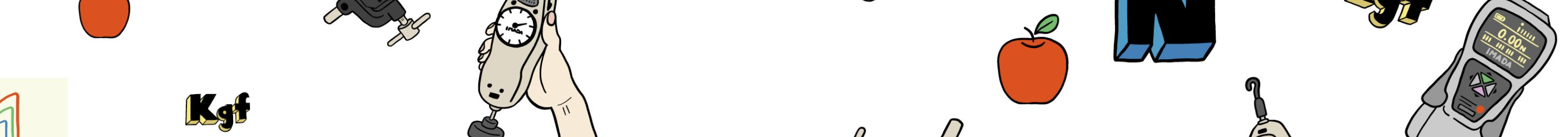# Measure this and that!## Experimental Column “Measure Power and the Strength with the Exercises Band”

Aug 28,2023

When you have a little time for some exercise, one of the simple solutions could be the band exercise. There is multiple information found on choosing the best load band that is most effective to the needs, and with the correct performance using suitable band. Choosing the exercise band, unlike the weights with “XX kg,” it is difficult to identify the actual intensity and the power applied for pulling the Bands during the exercises and how much of the true force is required for the practical exercises, as the pulling forces differ depending on the amount stretched. This article is an example of an experiment to evaluate the relationship between elongation and strength measurements of the exercise band.

## 1. Elongation and the Strength Relationships

We have evaluated the pulling force with the exercise band (Material: Latex, Width: 5cm), using a force gauge based on creating a similar situation as the rear pulldown movement to evaluate the elongation and the strength measured behind the neck as the image.

To start, the band with the force gauge fixed to the jig on one side and held above the head, the band length set at 15cm unstretched. Bend the elbows down for the band to stretch to 30cm, and the pulling force was measured. The result is as follows.

With the band used, the training intensity obtained was 19.8N * (≈2.019kgf) at the peak.

Knowing the value of 2.019kgf, is practical. So, we see the peak value, and then the relationship between the extension distance and the strength is measured. Instead of measurement in the actual training environment, the devices are used for graphing the elongation distance and strength relationship.

*N and kgf are the force Units, and 1kfg is the gravitational force of an object with a mass of 1kg subjected to standard gravitational acceleration. 5kg weight means that the Force 5kgf is exerted directly below (in reality, gravitational acceleration varies by region, causing some discrepancies). The equation 1kgf = 9.80665N is also valid.

The vertical axis represents “strength (Force)”, and the horizontal axis represents “extended distances”. The gripping distance at the start of the measurement was set to 15cm, the same as in the pulldown movement.

The result is a strength of 19.3N (≈1.968kgf) at an elongation distance of 15cm(150mm), which is very similar to the result obtained from the pulldown movement. The graph also shows that the strength increased significantly at the earlier stage of the measurement, and then strength and elongation distance increased gradually in proportion. Incidentally, the same trend was observed in the graph when bands of different hardness were measured.

Based on the measurement results, stretching the band firmly and extending it during the training is necessary to obtain high and adequate exercise strength. In addition, when training for a short extension distance, choosing a band stiffer with more load is an alternative practical training solution.

Training intensity is affected by the significant strength increase at the earlier stage of the band elongation. The intensity increases immediately after the elongation distance towards the middle, and the strength and elongation distance increase gradually and proportionally.

Now that the relationship between elongation and strength is identified, how, then, to adjust training intensity for the ideal exercise? For extending distances, there may be some limitations. It is possible to adjust using different band intensities, but there may be better options than purchasing many bands.

– Using Multiple Bands together

In general, the above methods seem practical for the strength increase.

But does it work? The graphs below show the results of the measurement.

– Using Multiple Bands together

Obviously, the strength increases with using multiple bands together. Checking the value at each distance, the power when using two bands together at once is roughly the sum of the two. This result increases the possibilities of training versatility when limited bands are available.

The strength of the band was also increased by holding the band shorter. Also, the stretching distance is increased during exercise, which may be an effective means to increase the intensity significantly. However, it may be necessary to note that the greater the load on the band itself, the greater the risk of the band breaking.

* The data obtained from these experiments is not for the recommendation how to use exercise bands.

## 3. Summary

In this article, we have measured the strength of exercise bands by force measurement. We hope there are some interesting discoveries from the data obtained. Of course, it may be challenging to know in-depth.

It is also tricky to understand the strength of each band without a specific measuring instrument. Still, we hope this article may give some information about the characteristics of exercise bands and help with a better understanding.

In this measurement, we used our force-measuring instrument, and based on the measurement data, the Graphs indicated “Force(N)→Strength(N)” on the vertical axis and the “Displacement(mm)→Distance extended(mm)” on the horizontal axis.